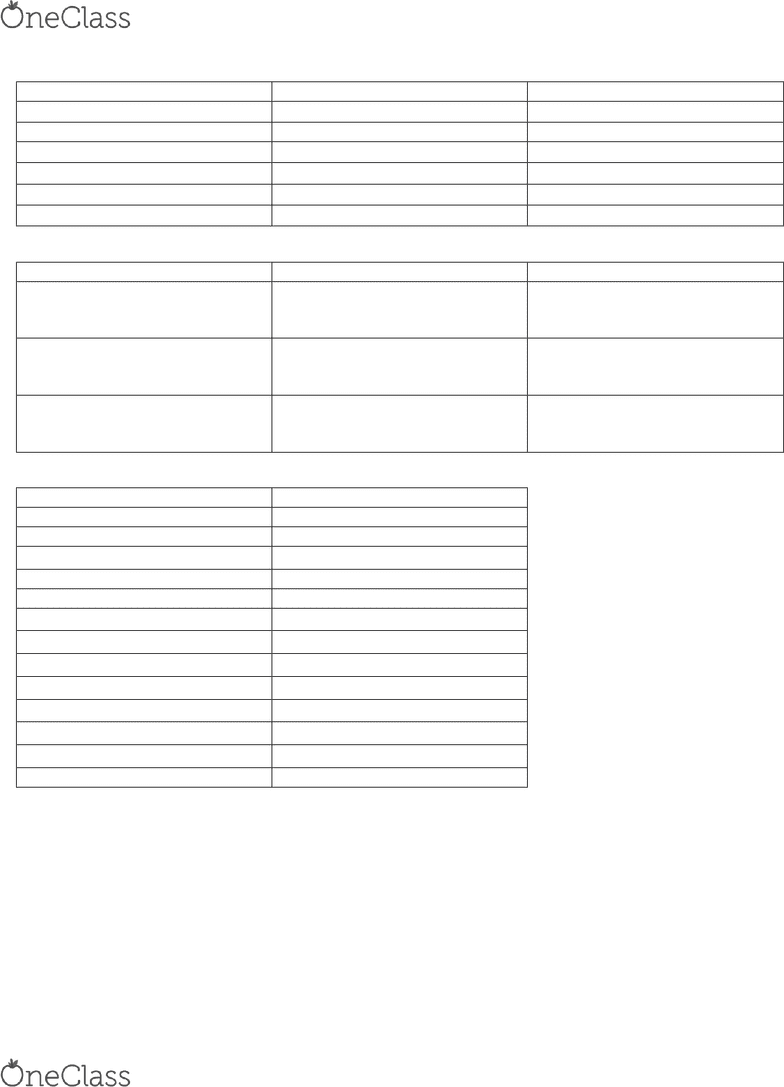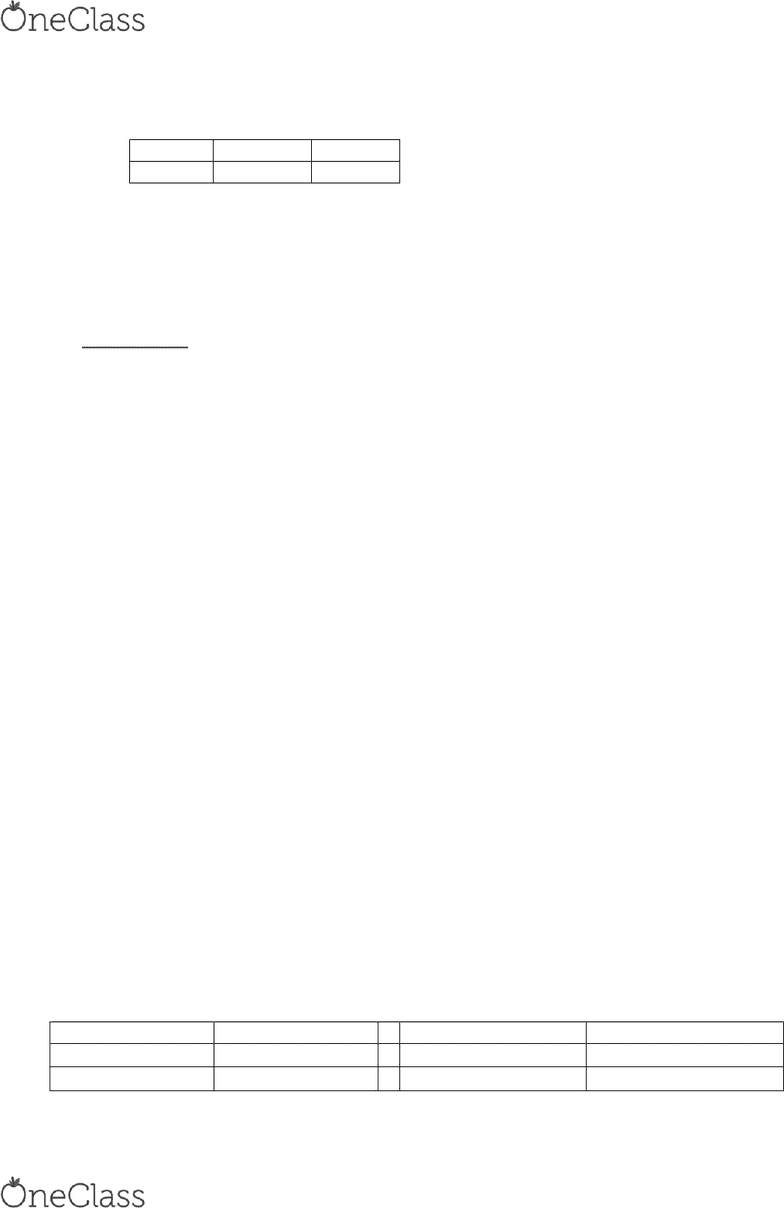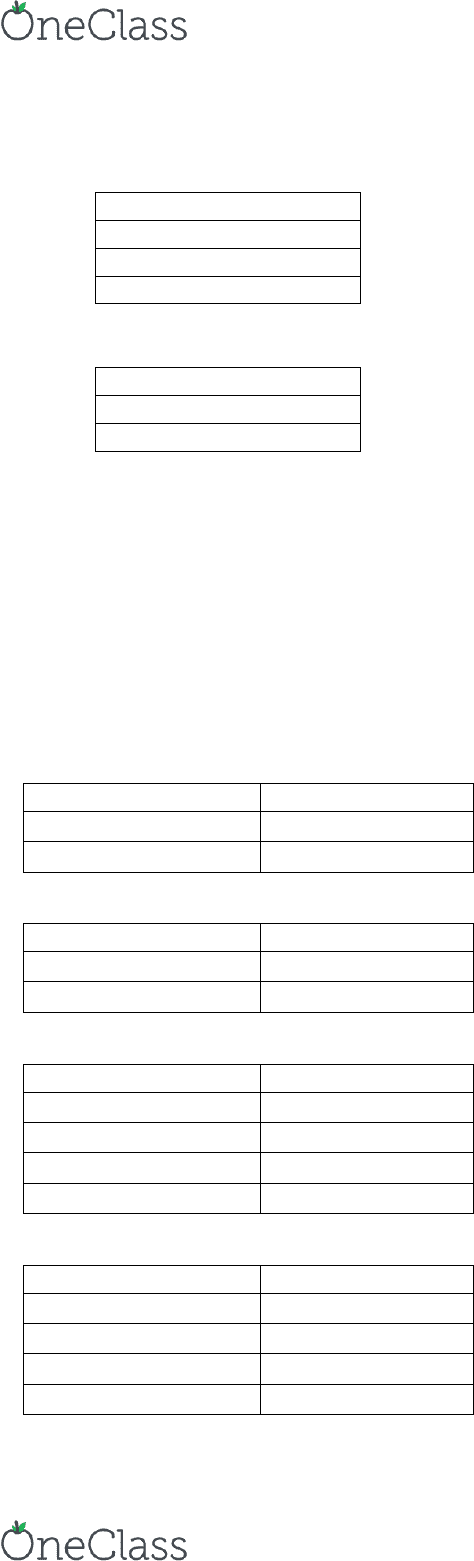Study Guides (400,000)
CA (150,000)
York (10,000)
ITEC (100)
Final

# ITEC 2600 Final: Summary of Characters, Commands, and Functions (Final Exam Study Guide)

Department
Information Technology
Course Code
ITEC 2600
Professor
Marshall Walker
Study Guide
Final

This preview shows pages 1-2. to view the full 8 pages of the document.BASIC OPERATIONS:
OPERATION
SYMBOL
EXAMPLE
+
5 + 3
Subtraction
5 3
Multiplication
*
5 * 3
Right Division
/
5 / 3
Left Division
\
5 \ 3 = 3 / 5
Exponential
^
5 ^ 3 = 53 = 125
COMMAND
DESCRIPTION
EXAMPLE
format short
4 decimal digits
>> 290 / 7
ans =
41.4286
format long
>> 290 / 7
ans =
41.428571428571431
format bank
Two decimal digits
>> 290 / 7
ans =
41.43
COMMAND
DESCRIPTION
sqrt (x)
square root
nthroot(x , n)
Nth real root
exp (x)
ex
abs (x)
absolute value
log (x)
natural log (base e)
factorial (x)
x!
sin (x)
cos (x)
tan (x)
sind (x)
sine (x in degrees)
cosd (x)
cosine (x in degrees)
tand (x)
tangent (x in degrees)
round (x)
Round to the nearest integer
A variable is a name that is assigned a numerical value:
o Can read the variable (get its value)
o Can write to the variable (set its value)
o Case sensitive: an underscore is placed in place of a space (e.g. speed_of_light or speedOfLight)
Keywords: pi (π), inf/Inf (infinity)
find more resources at oneclass.com
find more resources at oneclass.com

Only pages 1-2 are available for preview. Some parts have been intentionally blurred.VECTORS AND MATRICES:
A matrix is used to store a set of values of the same type; every value is stored in an element.
o A matrix with m rows and n columns is called m x n; these are called dimensions. e.g. this is a 2x3 matrix
9
6
3
5
7
2
o The term array is used in MATLAB to refer generically to a matrix or a vector.
A vector is a special case of matrix in which one of the dimensions is 1:
o A row vector with n elements is 1 x n, e.g. 1 x 4
o A column vector with m elements is m x 1, e.g. 3 x 1
A scalar is an even more special case; it is 1 x 1, or in other words, just a single value.
Direct Method: put the values you want in square brackets, separated by either commas or spaces.
o >> v = [1 2 3 4]
v =
1 2 3 4
Colon operator: iterates through values in the form first : step : last. E.g. 4 : -1 : 1 creates [4 3 2 1]
The function linspace creates a linearly spaced vector; linspace(x,y,n) creates a vector with n values in the
inclusive range from x to y
o E.g. linspace(4,7,3) creates a vector with 3 values including the 4 and 7 so it returns [4 5.5 7]
Built-in functions:
o rand (n) creates an nxn matrix of random reals
o rand(n,m) creates an nxm matrix of random reals
o randi([range], n, m) creates an nxm matrix of random integers in a specified range
o min the minimum value
o max the maximum value
o sum the sum of the elements
o prod the product of the elements
length(vec) returns the # of elements in a vector
length(mat) returns the larger dimension (row or column) for a matrix
size returns the # of rows and columns for a vector or matrix
numel returns the total # of elements in a vector or matrix
CHAPTER 3: STRINGS
A string is an array of characters
Create a string by typing characters within single quotes 
Elementwise operations on arrays:
Symbol
Description
Symbol
Description
.*
Multiplication
./
Right Division
.^
Exponentiation
.\
Left Division
Dot product [dot(a,b)]
find more resources at oneclass.com
find more resources at oneclass.com

Unlock to view full version

Only pages 1-2 are available for preview. Some parts have been intentionally blurred.WEEK 4: INPUT
The input function does two things: prompt the user and reads in a value.
o variablename = iputpropt strig
Conversion characters (fprintf)
%d integers
%f floats(real numbers)
%c single characters
%s strings
Formatting output:
\n newline character
\t tab character
\\ to print one slash
WEEK 5: 2D PLOTS
plot (x,y)
plot ,, lie specifier, PropertNae, PropertValue
o x is a vector
o y is a vector
o line specifiers specifiers that define the type and color of the line and markers.
o PropertyName and PropertyValue properties with values that can be used to specify the line width,
ad arkers size ad edge, ad fill colors.
Line Style
Specifier
Solid (default)
-
Dashed
--
Line Style
Specifier
Dotted
:
Dash-dot
-.
Line Color
Specifier
red
r
green
g
blue
b
cyan
c
Line Color
Specifier
magenta
m
yellow
y
black
k
white
W
find more resources at oneclass.com
find more resources at oneclass.com